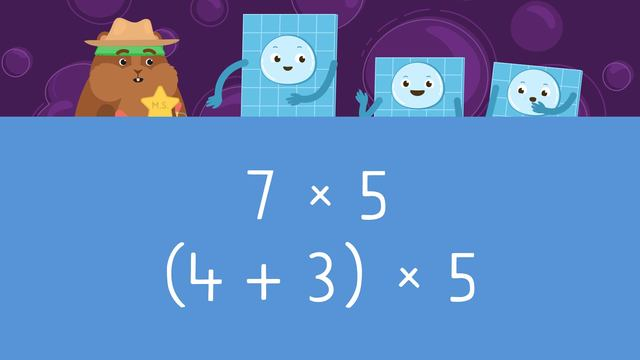# Distributive Property of MultiplicationRating

Ø 5.0 / 2 ratings

The authorsTeam Digital
Distributive Property of Multiplication
CCSS.MATH.CONTENT.3.OA.B.5

## Basics on the topicDistributive Property of Multiplication

### In This Video on 3rd Grade Distributive Property

Mr. Squeaks needs to use the distributive property of multiplication to help Big ‘Arry find out how many squares he has in all. After he learns what does distributive property mean, Mr. Squeaks learns how to do distributive property, and solves Big ‘Arry’s problem. At the bottom, you will find a distributive property worksheet!

### What is Distributive Property of Multiplication?

What is the distributive property of multiplication? The distributive property of multiplication is when you break down one factor into smaller parts, to multiply the broken down parts by the other whole factor, and then adding them together to get the product of the original factor pair. This is the distributive property definition math. Below is a quick example of distributive property.Now you have the answer to ‘what is the distributive property in math’, why is it useful? It is useful because it can help break down more challenging multiplication problems into smaller ones.

### Distributive Property Examples

Let’s have a go at distributive property math! Below, we have the factor pair four and six.First, we will break down the six into three and three.Then, we will distribute the four to be threes, to make four times three and four times three.Next, we find the product of both smaller factor pairs. Since they are both four times three, the product for both is twelve, so write the solution.Finally, add the two products together. Twelve plus twelve gives a sum of twenty-four. The product of four times six is twenty-four!### Distributive Property Multiplication Summary

To use the distributive property of multiplication, remember:

• Break down one of the factor pairs
• Distribute the whole factor to the broken down factor pairs
• Multiply both smaller factor pairs
• Add the product of both to find the product of the original factor pairUnderneath, you will find more practice examples of distributive property and a distributive property of multiplication worksheet.